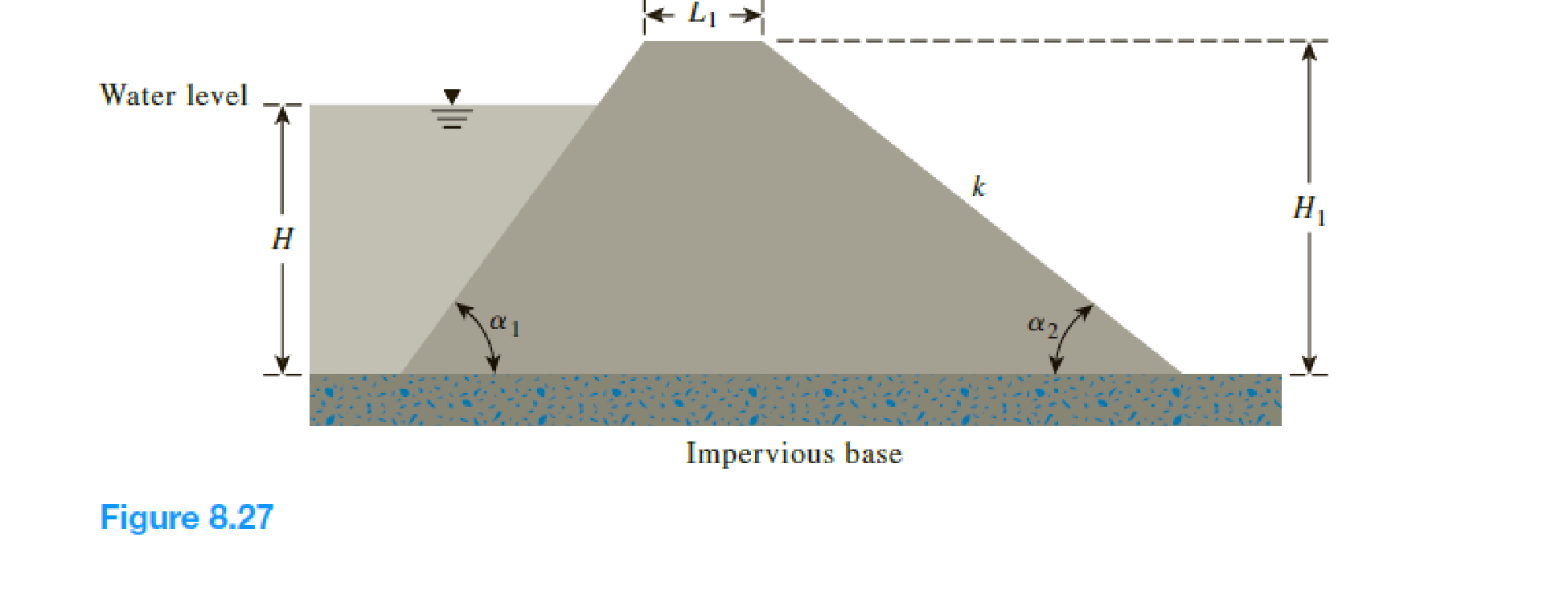Chapter 8, Problem 8.8PPrinciples of Geotechnical Enginee...

9th Edition
Braja M. Das + 1 other
ISBN: 9781305970939

Solutions

Chapter
SectionPrinciples of Geotechnical Enginee...

9th Edition
Braja M. Das + 1 other
ISBN: 9781305970939
Textbook Problem

An earth dam is shown in Figure 8.27 Determine the seepage rate, q, in m3/day/m length. Given: α 1 = 35 ° , α 2 = 40 ° , L1 = 5 m, H = 7 m, H1 = 10 and k = 3 × 10–4 cm/sec. Use Schaffernak’s solution.To determine

Find the seepage rate q, in m3/day/m length using Schaffernak’s solution.

Explanation

Given information:

The given angle α1 is 35°.

The given angle α2 is 40°.

The height of the water level H is 7 m.

The height of the earth dam H1 is 10 m.

The length of earth dam top L1 is 5 m.

The hydraulic conductivity of the permeable soil layer k is 3×104cm/sec.

Calculation:

Determine the value of Δ using the relation.

Δ=Hcotα1

Substitute 7 m for H and 35° for α1.

Δ=7cot35°=7×cos35°sin35°=10m

Determine the distance d value using the relation.

d=H1cotα2+L1+(H1H)cotα1+0.3Δ

Substitute 10 m for H1, 40° for α2, 5 m for L1, 7 m for H, 35° for α1, and 10 m for Δ.

d=10cot40°+5+(107)cot35°+0.3×10=10cos40°sin40°+5+(107)cos35°sin35°+0.3×10=24.2m

Determine the length L value using the relation

Still sussing out bartleby?

Check out a sample textbook solution.

See a sample solution

The Solution to Your Study Problems

Bartleby provides explanations to thousands of textbook problems written by our experts, many with advanced degrees!

Get Started

For Problem 19.27, calculate the probability distribution and plot the probability distribution curve.

Engineering Fundamentals: An Introduction to Engineering (MindTap Course List)

What is a business rule, and what is its purpose in data modeling?

Database Systems: Design, Implementation, & Management

What is data independence, and why is it lacking in file systems?

Database Systems: Design, Implementation, & Management

Explain issues surrounding payment for assistive technologies.

Enhanced Discovering Computers 2017 (Shelly Cashman Series) (MindTap Course List)

What causes most electric shock in the welding industry?

Welding: Principles and Applications (MindTap Course List)